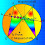## Tuesday, April 7, 2009

### Problem 23: Right triangle, Altitude, Inradius

Proposed ProblemSee complete Problem 23 at:
gogeometry.com/problem/p023_right_triangle_incircles.htm

Level: High School, SAT Prep, College geometry

1.Under the standard nomenclature, AD = c*sin(C)= c*c/b=c^2/b while BD=c*cos(C)= ac/b ----(1)
= (c^2/b*ac/b)/(c^2/b + ac/b + c)
= ac^2/b(a+b+c)
Similarly, r2 = ca^2/b(a+b+c)
and r = ac/(a+b+c)
So,r1+r2+r=ac^2/b(a+b+c)+ca^2/b(a+b+c)+ac/(a+b+c)
= ac/b = BD by equation (1)
Ajit: ajitathle@gmail.com

2.The statement that r=ac/(a+b+c) is incorrect; the numerator should be 2ac.

However, it is easier to show that r=a+b-c and use that to obtain the desired result.

3.Prof Elie Achkar (Lebanon)

BD + DC = BC + 2r1 in the triangle BDC
AD + BD = AB + 2r2 in the triangle BDA
AB + BC = AC + 2r in the triangle ABC

then :
BD+DC+AD+BD+AB+BC = 2r1 + 2r2 + 2r
THEN :
2BD = 2(r1 + r2 +r )
follow : BD = r1 + r2 + r

4.Inradius = Tr. Area/Semisum = (ac/2)/[(a+b+c)/2]Hence r = ac/(a+b+c)
So then why is the statement incorrect?

5.Dear Joe = Ajit:
I agree, your statement r = ac/(a+b+c) is correct.

6.How is it possible that
BD + DC =BC + 2r1
.................
.................

Please tell me the condition no

7.In a right triangle ABC (right angled at B)
r=s–b,is well-known.

Easily remembered as :
(or)
in-diameter=perimeter–twice the hypotenuse

So 2(r + r1 + r₂)
=2BD+AC–AC
=2BD

Hence r+r1+r₂=BD

8.If O1, O2 and O are the centers of circles with radius r1,r2 and r then

r1 = rc/b and r2 = ra/b since Tr.s AO1C, CO2B and AOC are similar

So r+r1+r2 = r (a+b+c)/b ....(1)

By writing the area of the right Tr. in 2 ways

BD. b = r(a+b+c)

So from (1) r+r1+r2 = BD

Sumith Peiris
Moratuwa
Sri Lanka